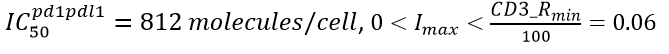Blog

## Estimation of Imax and IC50 for PD1-PDL1 inhibition of T cell proliferation

Oleg Demin
April 18, 2022

Introduction

PD1 and PD-L1 are surface molecules located on T cells and cancer cells (and APC), correspondently. When Immunological Synapse (IS) is formed between the cells PD-L1 is able to bind PD1, thereby stimulating inhibitory signaling in T cells which results in suppression of T cell proliferation. There were several studies ([PMID: 12517932], [PMID: 11015443], [PMID: 11857337]) where authors measured proliferating response of T cells in vitro as function of varying amount of PD-L1 immobilized on the surface of the beads/microspheres added to the T cells. Basing on the experimental results we can identify total amount of PD-L1 located on the all beads/microspheres added to the test tube corresponding to half maximal inhibition of T cell proliferation. But the value does not allow us to understand how many PD1-PDL1 complexes should be formed in IS to provide half maximal suppression of T cell proliferation.

In this study we will estimate IC50 and Imax characterizing effect of PD1-PDL1 complex on T cell proliferation. For the purposes we will use in vitro experimental data measured for human CD4 T cells published in [PMID: 12517932].

Description of experimental data utilized for IC50 and Imax estimation

To estimate IC50 and Imax of PD1-PDL1 complex affecting T cell proliferation we will use in vitro experimental data measured for human CD4 T cells published in [PMID: 12517932]. Fig. 1 represents dependence of CD3 proliferative response on total amount of PD-L1 added digitized from Fig 2A of the paper.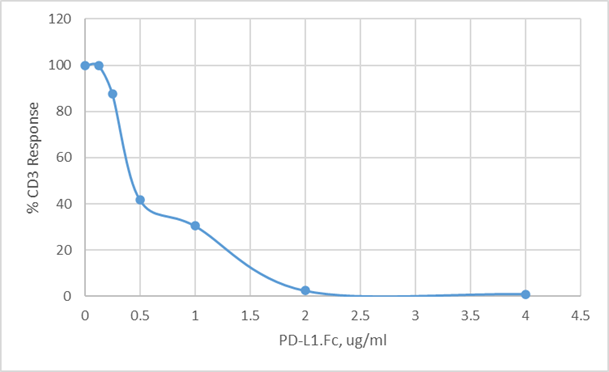Fig. 1. Dependence of CD3 proliferative response on total amount of PD-L1 added to the test tube digitized from Fig 2A of the paper [PMID: 12517932]

Description of experimental design was taken from sub-sections “Abs and fusion proteins” and “T cell assays” of “Materials and methods” section (clauses (i), (ii), (iii)) and legend for Fig. 2 (clause (iv)) of [PMID: 12517932]:

(i) “Construction of PD-L1.Fc (human (hu)PD-L1-murine (mu)IgG2a)”. Here, PD-L1.Fc is fusion protein of human PD-L1 and murine IgG2.

(ii) “Human CD4 T cells were puri?ed … from peripheral blood lymphocytes. Tosyl-activated magnetic microspheres (Dynal Biotech, Great Neck, NY) were coated with anti-CD3 Ab (0.25–3 ug/107 microspheres), and PD-L1.Fc (0.5–4 ug/107 microspheres)…”

(iii) “Protein-coated microspheres were added to puri?ed CD4 T cells (105 cells/well) in ?at-bottom 96-well microtiter plates at a ratio of 1:1; 72 h after culture initiation, plates were pulsed with 1 uCi tritiated thymidine/well and incubated for a 6- to 16-h period.”

(iv)  “Puri?ed CD4 T cells (105 cells/well) were activated with microspheres coated with a ?xed concentration of anti-CD3 Ab (1 ug/107 microspheres) and increasing concentrations of PD-L1.Fc …. For comparison purposes, values are represented as the percentage of anti-CD3 Ab response, where 100% represent cpm obtained when cells were activated with anti-CD3/murine IgG-coated microspheres.”

Workflow for estimation of IC50 and Imax basing on experimental data

Estimation of the parameters will be performed in several consecutive steps:

1. Calculation of number of PD-L1.Fc attached to microspheres
2. Calculation of number of PD1-PDL1 complexes in IS formed by T cells and microspheres with different PD-L1 density
3. Estimation of IC50 and Imax basing on values calculated in clauses (1) and (2)

Calculation of number of PD-L1.Fc attached to microspheres

Calculation of number of PD-L1.Fc in 1 ug

Basing on clause (i) of section “Description of experimental data utilized for IC50 and Imax estimation” fusion protein PD-L1.fc consists of human PD-L1 and murine IgG2. Let us calculate Molecular Weight (MW) of PD-L1.Fc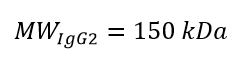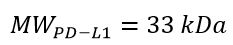[https://www.genscript.com/protein/Z03425-PD_L1_His_Human.html]

As a result, one obtains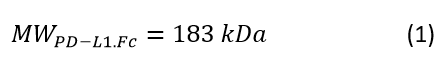Taking (1) into account we can calculate how many umoles are in 1 ug of PD-L1.Fc:

(umoles of PD-L1.Fc in 1 ug) = (1e6 umoles)*(1 ug)/(1.8e11 ug) = 5.6e-6 umoles     (2)

Recalculate (2) in number of molecules:

(number of PD-L1.Fc in 1 ug) = (5.6e-6 umoles)*(6.03e17 molecules/umole) = 3.4e12 molecules

(3)

Calculation of number of PD-L1.Fc per microsphere

Basing on clause (ii) of section “Description of experimental data utilized for IC50 and Imax estimation” density of PD-L1.Fc on microspheres varies in the range 0.5–4 ug/(107 microspheres).

Taking into account number of PD-L1.Fc molecules in 1 ug we can calculate number of PD-L1.Fc per microsphere for density 1 ug/(107 microspheres) as follows:

(number of PD-L1.Fc per microsphere for density 1 ug/(107 microspheres)) = (number of PD-L1.Fc in 1 ug)/ (107 microspheres) = 3.4e12 molecules/(107 microspheres) = 3.4e5 molecules/microsphere                                                                                                              (4)

In similar way we can calculate range of number of PD-L1.Fc per microsphere as follows:

{0.5–4 ug/(107 microspheres)} ?{1.7e5 – 1.36e6 molecules/microsphere }          (5)

Calculation of number of PD1-PDL1 complexes in IS formed by T cells and microspheres with different PD-L1 density

Equations used to estimate number of PD1-PDL1 complexes in IS

Let us consider rate law describing binding of surface molecules in IS derived in InSysBio Blog publication entitled as “Derivation of rate law describing binding of surface molecules in immunological synapse” (see Eqs (8)-(10) in https://insysbio.com/blog/derivation-of-rate-law-describing-binding-of-surface-molecules-in-immunological-synapse ). Designating number of free molecules of PD1 per T cell, number of free molecules of PD-L1 per microsphere and number of complexes PD1 with PD-L1 per IS formed by T cell and microsphere as pd1, pdl1 and pd1pdl1 we can rewrite rate law of binding PD1 with PD-L1 in IS as follows: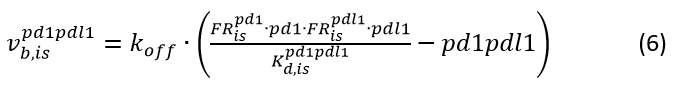Here,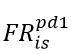and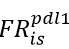represent fractions of cell surface corresponding to IS in T cells and microspheres, correspondently,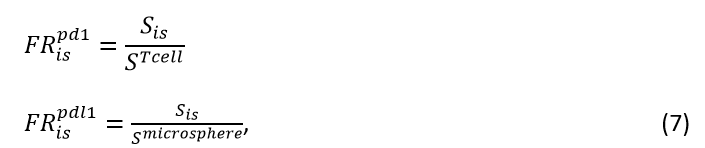and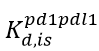represents 2D equilibrium dissociation constant multiplied by area of IS formed by T cell and microsphere: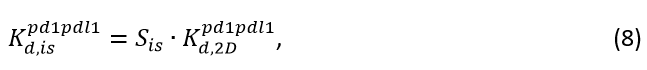Here, STcell, Smicrosphere, Sis stand for surface area T cell, microsphere and IS formed by T cell and microsphere.

To calculate number of PD1-PDL1 complexes in IS we will use assumption that the process of binding of PD1 to PD-L1 is in quasi equilibrium, i.e.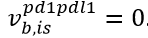. Taking into account conservation law for PD1, one obtains following system of algebraic equations: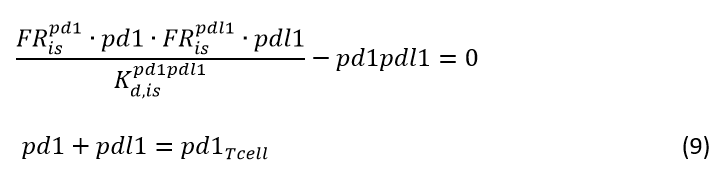Basing on the fact that number PD-L1 molecules immobilized on microspheres is much higher than number of PD1 molecules located on T cells we can assume that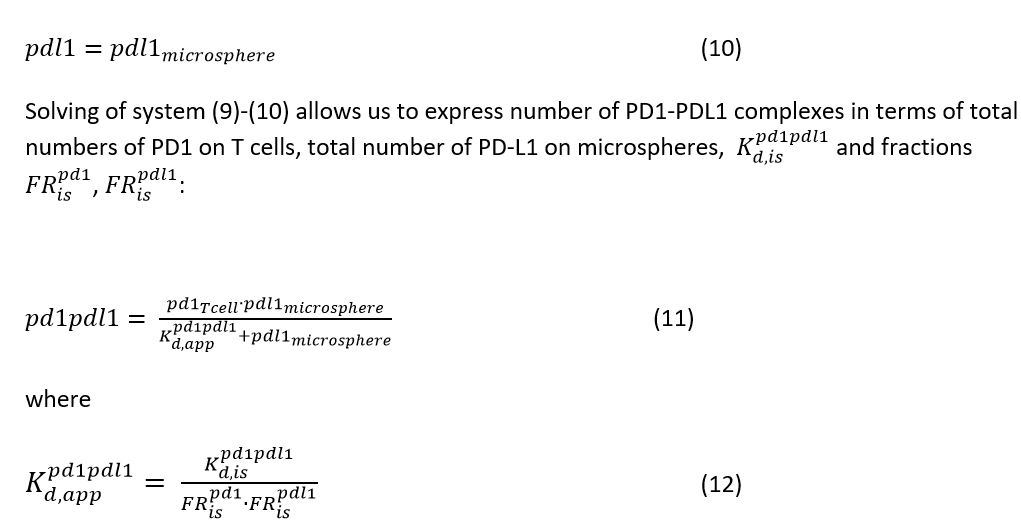Estimation of parameters appearing in equations (7)-(8), (11)-(12)

Let us estimate/calculate all parameters appearing in equations (7)-(8), (11)-(12).

ST cell= 152 um? Calculated from cell volume assuming spherical shape

Smicrosphere= 64 um2? Calculated from volume assuming spherical shape and assuming diameter = 4.5 um2 [https://www.thermofisher.com/order/catalog/product/11161D ]

Sis = 8.55 um2? basing in microsphere diameter = 4.5 um2 and estimates of contact area between T cells and beads of different diameter (see legend for Fig 3 in [PMID: 10411899])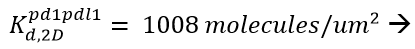calculated on the basis of Kd_3d_pd1pdl1 value measured in vitro as described in IRT [https://irt.insysbio.com/nav/# ]. Kd_3d_pd1pdl1 = 770 nM [PMID: 18585785, Fig 1C]

pd1T cell = 2.52e3 molecules/T cell ? [PMID: 31407460, Table 2B-C]                                (13)

pdl1microsphere is varied in accordance with (5)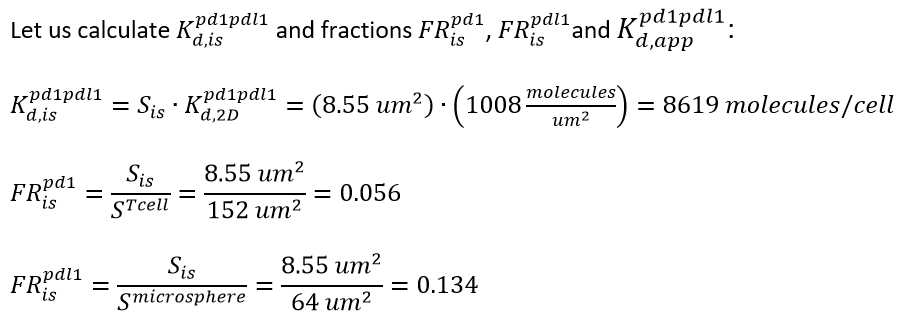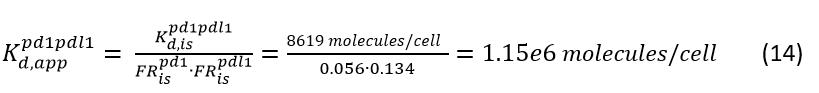Estimation of number of PD1-PDL1 complexes in IS corresponding to half maximal inhibition of T cell proliferation

Let us designate number of PD1-PDL1 complexes in IS between T cells and microsphere corresponding to half maximal inhibition of T cell proliferation as pd1pdl150.

To calculate the value of pd1pdl150 using Eq (11) we need to know how many molecules of PD-L1 was immobilized on microsphere (pdl150) allowing to reach half maximal inhibition in T cell proliferation. Number of PD1 molecules located on T cell is assumed to be fixed and equal to 2.52e3 molecules/T cell.

Fig 1 demonstrates that half maximal inhibition of T cell proliferation is observed at total concentration of fusion protein PD-L1.Fc in the test tube equal to approximately 0.4 ug/ml. Comparison of ranges of concentration of fusion protein indicated at the X axis of Fig 1 with ranges of PD-L1.Fc (0.5–4 ug/107 microspheres) (see clause (2) of section “Description of experimental data utilized for IC50 and Imax estimation”) we have concluded that

pdl150 = 1.6 ug/107 microspheres

In accordance with formula (5) we have come to following value of pdl150 expressed in molecules per microsphere:

pdl150 = 5.44e5 molecules/microsphere                                 (15)

Substituting values (13)-(15) into equation (11), one obtains: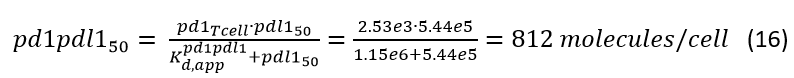Calculation of IC50 and Imax of PD1-PDL1 complexes in IS describing suppression of T cell proliferation

To calculate the values of IC50 and Imax we will use description of T cell proliferation entitled as “generation dependent proliferation” (see our poster for more details https://drive.google.com/file/d/15qMMMfC0dN_U1zBmtetRAKVNygSMdTke/view ). In accordance with the derivations presented at the poster time dependence of cell number is given by following formula: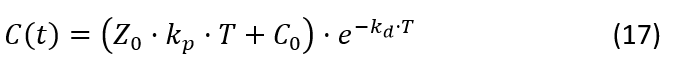Here C0, Z0 are initial conditions and kd, kp represent rate constant of T cell degradation and proliferation. T is time of measurement of T cell count after start of experiment. In accordance with clauses (3) and (4) of section “Description of experimental data utilized for IC50 and Imax estimation”, T = 72 hour.

Since PD1-PDL1 complex is inhibitor of T cell proliferation then in accordance with our general approach applied for description of regulatory effect of cell dynamic processes (see Eq (5) of InSysBio Blog publication entitled as “An approach to describe multiple effects of cytokines on cell dynamics processes” https://insysbio.com/blog/an-approach-to-describe-multiple-effects-of-cytokines-on-cell-dynamics-processes ), one obtains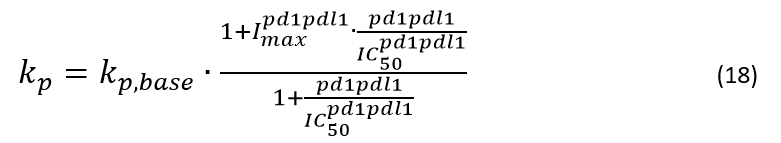Combining (17) and (18) and taking into account that in experiment presented at Fig 1 values of % CD3 Response represent the percentage of anti-CD3 Ab response, where 100% represent cpm obtained when cells were activated with anti-CD3/murine IgG-coated microspheres, i.e. microspheres without PD-L1, we can describe the experimental data as follows: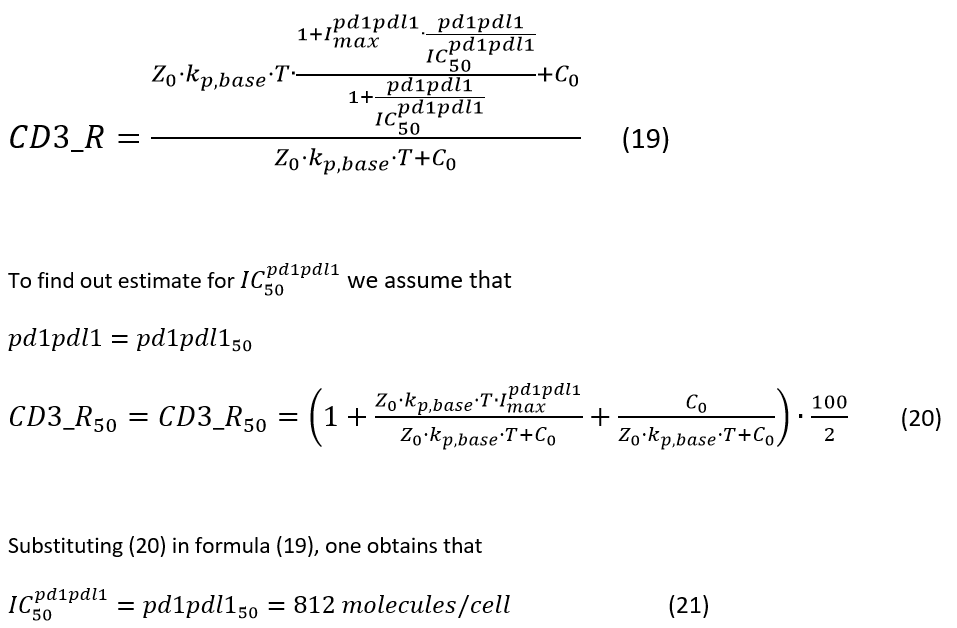In similar manner we can obtain following estimates for Imax: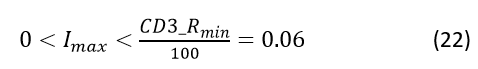Where CD3_Rmin = 6 is estimated from Fig 1 as value of % Cd3 Response at PD-L1.Fc = 4 ug/ml

Conclusion

Basing on previously derived rate law describing binding of surface molecules in immunological synapse and in vitro experimental data describing effect PD1-PDL1 complex on T cell proliferation, we have estimated IC50 and Imax quantifying the suppression effect of PD1-PDL1 complex as